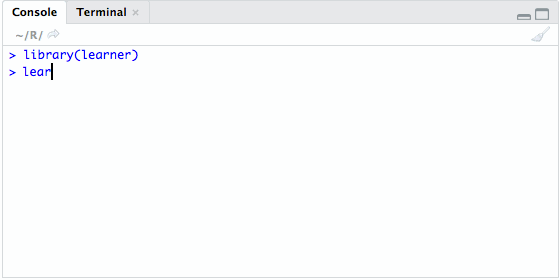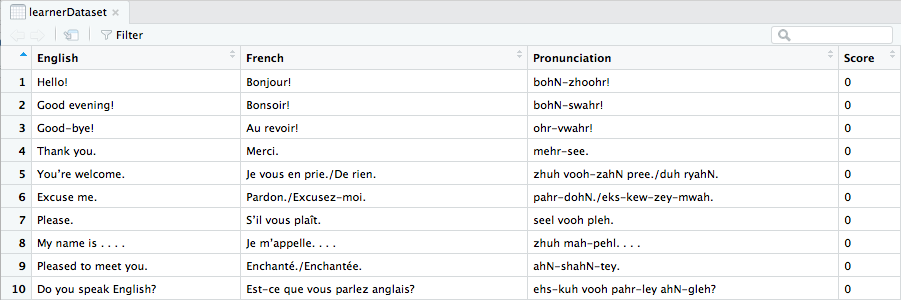# Introducing {learner}, My First Package!

The {learner} package lets you use the R console as an interactive learning environment in order to memorize any dataset you want. Its main goal is to put foreign language vocabulary learning in the R workflow, so R can also be used to study languages or anything related to flashcards.

The gif below shows how to use {learner} in the R console.### ** Update, March 24th, 2018 **

The {learner} package is now called {polyglot}. See this blog post for more information.

## My inspirations

I am a big fan of Anki, a spaced repetition program which priorities the flashcards you need to study. It’s a very effective application to memorize foreign vocabulary or anything you want to. And it’s free and open source.

As Anki is written in Python, I wondered if a similar program exists in the R community. The closer I could find was the impressive {swirl} package, which teaches you R programming and data science interactively in the R console. Sadly, {swirl} is not built to study flashcards. So to fill this gap, I decided to develop the {learner} package (my first package!).

## How {learner} works

The {learner} package is built in only 3 functions. I will present them now one by one.

Let’s begin by looking at the first and longer function, called learn, which launches the interactive learning environment.

learn <- function() {
message("| Welcome to learner!")
message("| Please choose a dataset to study, or type 0 to exit.")
if(!exists("learnerStartTime")) {
learnerStartTime <- Sys.time()
assign("learnerStartTime", learnerStartTime, envir = .GlobalEnv)
}
learnerDatasetPath <- system.file("extdata/", package = "learner")
learnerDatasetName <- select.list(list.files(path = paste0("", learnerDatasetPath, "")))
learnerDatasetAbsolutePath <- paste0("",learnerDatasetPath,"", learnerDatasetName,"")
assign("learnerDatasetAbsolutePath", learnerDatasetAbsolutePath, envir = .GlobalEnv)
if (learnerDatasetName == "") {
learnerExit() # 0 selected, exit the learning session
} else {
learnerDataset <- read.csv(paste0("",learnerDatasetAbsolutePath,""), stringsAsFactors = FALSE)
assign("learnerDataset", learnerDataset, envir = .GlobalEnv)
# Check if the dataset has at least two colomns
if (ncol(learnerDataset) < 2) {
message("| WARNING: Your dataset doesn't have two colomns.")
message("| Learner needs two-colomns datasets to work correctly.\n")
return(learn())
} else {
learnerDataset[,1] <- as.character(learnerDataset[,1])
learnerDataset[,2] <- as.character(learnerDataset[,2])
assign("learnerDataset", learnerDataset, envir = .GlobalEnv)
}
# Add numerical Score variable if not exists
if (any(names(learnerDataset) == "Score")) {
message(paste("|", learnerDatasetName,"selected, with", nrow(learnerDataset),"rows."))
message("| Continuing learning session...\n")
} else {
learnerDataset$Score <- rep(as.numeric(0), nrow(learnerDataset)) assign("learnerDataset", learnerDataset, envir = .GlobalEnv) write.csv(learnerDataset, file = paste0("", learnerDatasetAbsolutePath, ""), row.names = FALSE) message(paste("|", learnerDatasetName,"selected, with", nrow(learnerDataset),"rows.")) message("| New learning session launched...\n") } # If NAs exist in the Score variable, replace by 0 learnerDataset$Score[is.na(learnerDataset$Score)] <- 0 assign("learnerDataset", learnerDataset, envir = .GlobalEnv) write.csv(learnerDataset, file = paste0("", learnerDatasetAbsolutePath, ""), row.names = FALSE) return(learnerQuestion()) } invisible() } This function initializes the interactive environment by proposing to select a CSV file from the inst/extdata/ directory. The function reads the selected dataset and adds a numeric variable, named Score, if not already existing. It also replaces any existing missing values by 0 from the Score variable. It means that you can add new rows in the CSV file at any time you want. Finally the function returns to the learnerQuestion function, or to the learnerExit function if 0 is typed. When the dataset is chosen, you will have a data.frame named learnerDataset in your Global Environment. If it was for instance the “French_30_Basic_expressions.csv” dataset that has been selected, the data will look like a three-sided flashcards with a question, an answer and an hint/example (here pronunciation):center Now, let’s look at the second function, named learnerQuestion. learnerQuestion <- function() { learnerDataset <- read.csv(paste0("",learnerDatasetAbsolutePath,""), stringsAsFactors = FALSE) message(paste("| Question:", learnerDataset[1,1],"")) switch(menu(c("Show answer", "Hard", "Good", "Easy", "Hint/Example", "Back to menu")) + 1, return(learnerExit()), message(paste0("| Answer: ", learnerDataset[1,2], "\n")), learnerDataset$Score <- learnerDataset$Score + 1, learnerDataset$Score <- learnerDataset$Score + 2, learnerDataset$Score <- learnerDataset$Score + 4, if (names(learnerDataset) != "Score") { message(paste("| Hint/Example:", learnerDataset[1,3],"\n")) } else { message(paste("| No Hint/Example in this dataset.\n")) }, return(learn())) learnerDataset <- learnerDataset[order(learnerDataset$Score), ]
assign("learnerDataset", learnerDataset, envir = .GlobalEnv)
write.csv(learnerDataset, file = paste0("", learnerDatasetAbsolutePath, ""), row.names = FALSE)
invisible()
return(learnerQuestion()) # create loop
}

This function reads the selected dataset and print the first row of its first column, i.e. the question. Then it presents to the user a menu, which gives him multiple choices. According to the choice made by the user, the function gives a score point, i.e. Hard = +4 points, Good = +2 points, Easy = +1 point. The menu also proposes to show the answer (to print the 2nd column of the row), to give a hint/example (to print the 3rd column of the row), or to go back to the main menu. Finally, the function reorders the dataset in order to get the lower points score in its first row and returns the function once again.

Yes, I agree, the score algorithm is very basic. I will probably improve it in the next versions of the package. If you want to contribute or suggest any improvements, don’t hesitate to make a Pull request or to contact me.

Finally, let’s look at the learnerExit function.

learnerExit <- function() {
learnerEndTime <- Sys.time()
learnerTimer <- as.numeric(learnerEndTime - learnerStartTime, units = "mins")
if(round(learnerTimer) <= 1) {
message(paste("| Learning session time:", round(learnerTimer),"minute."))
} else {
message(paste("| Learning session time:", round(learnerTimer),"minutes."))
}
message("| Learning score saved. Cleaning Global Environment...")
if(exists("learnerDataset")) {
rm(learnerDataset, envir = globalenv())
}
if(exists("learnerDatasetAbsolutePath")) {
rm(learnerDatasetAbsolutePath, envir = globalenv())
}
if(exists("learnerStartTime")) {
rm(learnerStartTime, envir = globalenv())
}
message("| Leaving learner now. Type learn() to resume.")
invisible()
}

This function calculates the learning session time and print it. Then, it cleans the Global Environment if needed and displays a farewell message.

Happy learning!